### Visualising Solid Shapes - Worksheets

CBSE Worksheet-1
CLASS –VII Mathematics (
Visualising Solid Shapes)

1. Give two examples of plane figures.
2. Define the net of a solid.
3. Identify the nets which can be used to make cubes.
a.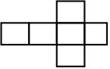b.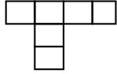4. Can this be a net for a die? Explain your answer?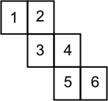5. A box is in the shape of a cuboid. If its length, breadth and height are 50 cm, 20 cm and 15 cm respectively, find its surface area.
6. How many wooden cubical blocks of edge 12 cm can be cut from another cubical block of wood of edge 3 m and 60 cm?
7. Write the number of faces, edges and vertices in the solids given below.
a. Cube b. Pyramid
8. Match these two dimensional figures with their names.
 i)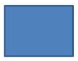a) Triangle ii)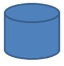b) Rectangle iii)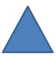c) Trapezium Iv)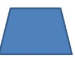d) Cylinder
CBSE Worksheet-1
CLASS –VII Mathematics (
Visualising Solid Shapes)
 i)a) Rectangle ii)b) Cylinder iii)c) Triangle Iv)d) Trapezium